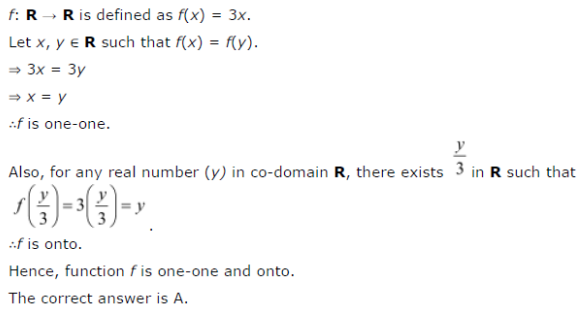# Let f : R → R be defined as f(x) = 3x Choose the correct answer:

Let f : R → R be defined as f(x) = 3x Choose the correct answer:
(A) f is one-one onto
(B) f is many-one onto
( C) f is one-one but not onto
(D) f is neither one-one nor onto